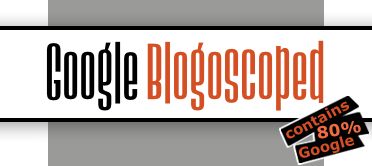### Forum

 ZimTuesday, November 13, 200714 years ago • 4,486 views Search something at google images.Then, put the following in the address bar, and hit enter:javascript:R=0; x1=.1; y1=.05; x2=.25; y2=.24; x3=1.6; y3=.24; x4=300; y4=200; x5=300; y5=200; DI= document.images; DIL=DI.length; function A(){for(i=0; i

Forum home